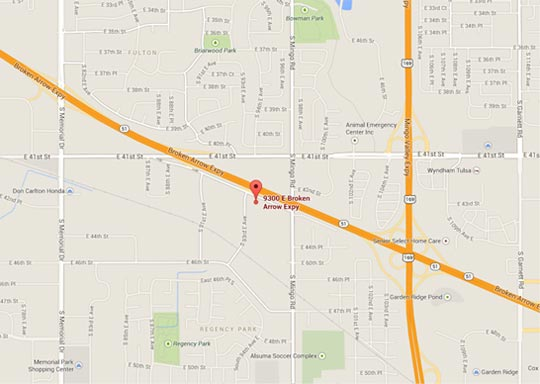Posts By Month:

# Blog | How Many Gallons of Water are in your Pool?

To figure the gallons of water in your pool you need to know what the surface area of you pool is. To determine this you need to know the length and width of the pool. Then you need to know the average depth. Then lastly you need to know how many gallons are in a cubic foot. The formula looks like this...

Length x Width x Average Depth x 7.48 = Gallons of water in your pool

Here is an example:

Lets say you have a pool that is a 18' x 36' rectangle and it goes from 3.5' to 6' with a sloping floor. First you multiply 18' x 36'= 648 sq. ft. Then you find the average depth, the simple way is to add the shallow end and deep end depth and divide by two. That is 3.5' + 6'= 9.5' then 9.5' / 2= 4.75 So now you multiply 648 x 4.75= 3078 now we multiply that number by 7.48 to get the total volume. So 3078 x 7.48= 23,023.44 gallons.

If you have a pool that is a free form shape with various widths and lengths you can measure across the pool 4 or 5 times and add those numbers then divide by the amount of times you measured. For instance if you measure 13' + 15' + 18' + 14.5' + 19.5' = 80 / 5 = 16' average width do the same with the length and then multiply those two numbers. This will give you a more accurate total surface area. Also for a more accurate depth you can measure the depth four or five times from shallow to deep and add those numbers then divide by times measured to get a more accurate depth. Then lastly again multiply x 7.48 (gallons in a cubic ft.) .

An interesting note a gallon of water weighs around 8.35 lbs. So in our example above we would multiply total gallons x 8.35 to get the total weight of the water in the pool or 23,023.44 x 8.35 = 192,245.72 lbs.

Wow that is a lot of weight, another way to look at it is an average car weighs about 4000 lbs. so that is like having 48 automobiles (192,245 / 4000) stacked in your pool!

Topics: all , How Many Gallons of Water are in your Pool?

##Valuable Information Here!Free 8 page Informative design booklet!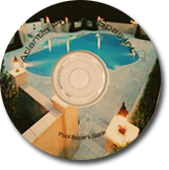## Our Mission Statement

Atlantis Pools & Spas Inc. will educate consumers about swimming pools, have the most highly trained personnel, build the absolute best swimming pools with the latest in technological features, and be aware that completing jobs on time is of importance to all for a mutually beneficial experience.

# Financing

We accept: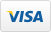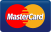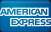For those interested in financing, we have 100% financing O.A.C. through HFS Financial. Apply online now for your Atlantis Pool! Click the secure link below.# Call Today(918)641-1100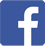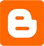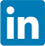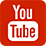9300 E. Broken Arrow Expressway, Ste D
Tulsa, Ok. 74145
Fax: (918) 641-1115

Please Fill out our project form to help us understand the scope of your project